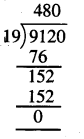# PSEB 4th Class Maths Solutions Chapter 4 Money (Currency) Ex 4.5

Punjab State Board PSEB 4th Class Maths Book Solutions Chapter 4 Money (Currency) Ex 4.5 Textbook Exercise Questions and Answers.

## PSEB Solutions for Class 4 Maths Chapter 4 Money (Currency) Ex 4.5

1. Divide the following amounts :

Question 1.
₹ 160 ÷ 4
Solution: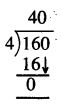∴ ₹ 160 ÷ 4 = ₹ 40Question 2.
₹ 475 ÷ 5
Solution: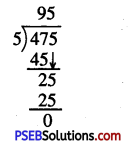∴ ₹ 475 ÷ 5 = ₹ 95

Question 3.
₹ 564 ÷ 12
Solution: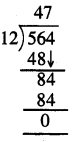∴ ₹ 564 ÷ 12 = ₹ 47

Question 4.
₹ 1248 ÷ 6
Solution: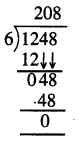∴ ₹ 1248 ÷ 6 = ₹ 208Question 5.
₹ 2665 ÷ 13
Solution: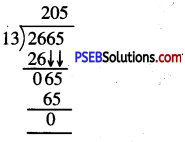∴ ₹ 2665 ÷ 13 = ₹ 205

Question 2.
The cost of 18 toy cars is ₹ 450. Find the cost of one toy car ?
Solution:
Cost of 18 toy cars = ₹ 450
Cost of 1 toy car = ₹ 450 ÷ 18
= ₹ 25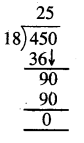Question 3.
The cost of 13 books is ₹ 936. Find the cost of one book.
Solution:
Cost of 13 books = ₹ 936
Cost of 1 book = ₹ 936 ÷ 13
= ₹ 72.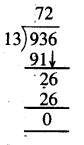Question 4.
The cost of a dozen oranges is ₹ 84. Find the cost of one orange.
Solution:
Cost of one dozen i.e. 12 oranges = ₹ 84
Cost of one orange = ₹ 84 ÷ 12
= ₹ 7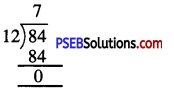Question 5.
An amount of ₹ 2848 is to be distributed among 16 children. What amount will each child get ?
Solution:
Amount to be distributed among 16 children = ₹ 2848
Amount received by each child = ₹ 2848 ÷ 16
= ₹ 178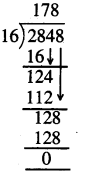Question 6.
A school received ₹ 9120 to buy uniforms for 19 children of class 4. How much amount did the school get for each uniform.
Solution:
Total amount received by the school = ₹ 9120
No. of children = 19
Amount of money school received for each uniform
= ₹ 9120 ÷ ₹ 19
= ₹ 480.